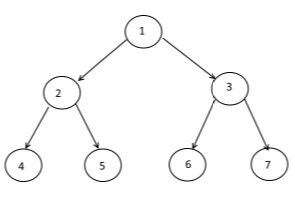# Level Order traversal - Implementation

suggest change

For example if the given tree is:Level order traversal will be

1 2 3 4 5 6 7

Printing node data level by level.

Code:

``````#include<iostream>
#include<queue>
#include<malloc.h>

using namespace std;

struct node{

int data;
node *left;
node *right;
};

void levelOrder(struct node *root){

if(root == NULL)    return;

queue<node *> Q;
Q.push(root);

while(!Q.empty()){
struct    node* curr = Q.front();
cout<< curr->data <<" ";
if(curr->left != NULL) Q.push(curr-> left);
if(curr->right != NULL) Q.push(curr-> right);

Q.pop();

}
}
struct node* newNode(int data)
{
struct node* node = (struct node*)
malloc(sizeof(struct node));
node->data = data;
node->left = NULL;
node->right = NULL;

return(node);
}

int main(){

struct node *root = newNode(1);
root->left        = newNode(2);
root->right       = newNode(3);
root->left->left  = newNode(4);
root->left->right = newNode(5);
root->right->left  = newNode(6);
root->right->right = newNode(7);

printf("Level Order traversal of binary tree is \n");
levelOrder(root);

return 0;

}``````

Queue data structure is used to achieve the above objective.